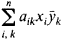# Hermitian Matrix

(redirected from Hermitian sequence)
Also found in: Dictionary.

## Hermitian matrix

[er′mish·ən ′mā·triks]
(mathematics)
A matrix which equals its conjugate transpose matrix, that is, is self-adjoint.
McGraw-Hill Dictionary of Scientific & Technical Terms, 6E, Copyright © 2003 by The McGraw-Hill Companies, Inc.
The following article is from The Great Soviet Encyclopedia (1979). It might be outdated or ideologically biased.

## Hermitian Matrix

(or self-adjoint matrix), a matrix coincident with its adjoint, that is, a matrix such that aik= āki, where ā is the complex conjugate of the number a. If the elements of a Hermitian matrix are real, then the matrix is symmetric. A Hermitian matrix has real eigenvalues λ1, λ2, …, λn and corresponds to a linear transformation in a complex n-dimensional space that reduces to stretchings by ǀλiǀ in n mutually perpendicular directions and reflections in the planes orthogonal to the directions for which λi < 0. A bilinear formwhose coefficients form a Hermitian matrix, is called a Hermitian form. Any matrix can be written in the form A1 + iA2, where A1 and A2 are Hermitian matrices, and in the form A ∪, where A is a Hermitian matrix and U is a unitary matrix. If A and B are Hermitian matrices, then A B is a Hermitian matrix if and only if A and B commute.